# Quiz 8: Benefitcost Analysis

Business

Given information: • Initial investment is \$5,000. • Annual maintenance cost is \$48,830. • Annual revenue is \$117,400. • Discount rate is 6%. • Time period is 5 years. Present equivalent worth of the annual cash flow can be obtained by using the following formula: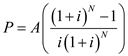…… (1)Where, P = Present equivalent worth A = Annual cash flow i = Interest rate N = Number of years Present equivalent worth: To obtain the benefit-cost ratio, equalize the present equivalent revenue of plan with its present equivalent cost. Equation (1) is used to convert the annual cash flow to present worth.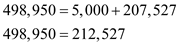Benefit-cost ratio: Benefit-cost ratio is as follows:Since the benefit-cost ratio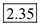is greater than 1, the project is economically feasible.

Given information: • Initial investment of the building is \$2,000,000. • Annual operating cost is \$200,000. • Annual revenue is \$500,000. • Rate of interest is 6%. • Time period is 8 years. Present worth of the annual cash flow can be obtained by using the following formula: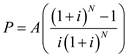…… (1)Where, P = Present worth A = Annual cash flow i = Interest rate N = Number of years Present worth of benefit: The present worth of benefit is calculated by using Equation - 1 as follows:Hence, the present worth of benefit is \$3,105,600 Present worth of cost: The present worth of cost can be calculated as follows: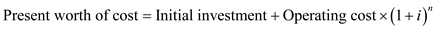…… (2)Present worth of cost: Using the Equation - 2, the present worth of cost is calculated as follows:Hence, the present worth of cost is \$2,318,800 Benefit cost ration: The benefit cost ratio can be calculated as follows: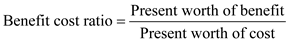…… (3)Benefit cost ratio: Using the Equation - 3, the benefit cost ratio can be calculated as follows:Hence, the benefit cost ratio is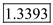. Hence, the answer is option (c)

The given information: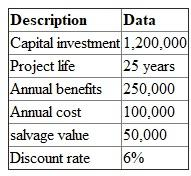Formula: The benefit - cost ratio: The benefit - cost ratio can be calculated by using the following formula: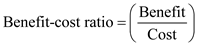…… (1)Present equivalent worth of the annual cash: Present equivalent worth of the annual cash flow can be calculated by using the following formula:…… (2)Where, P = Present equivalent worth A = Annual cash flow i = Interest rate N = Number of years The cost: The cost can be calculated as follows:Thus, the value of cost is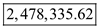Benefit: Benefit can be calculated as follows: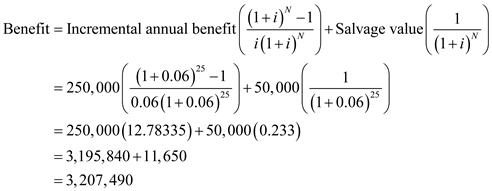Thus, the value of benefit is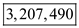Benefit - cost ratio: By using of equation (1), the benefit - cost ratio can be calculated as follows:Since, the value of benefit - cost ratio (1.29) is greater than 1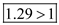, the project is economically feasible.

There is no answer for this question